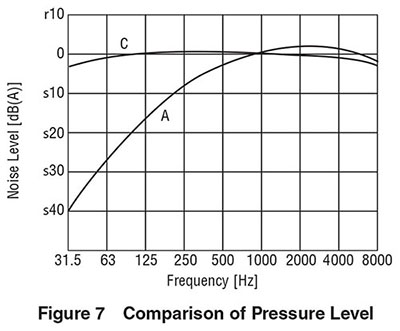Free Shipping for Online Orders. Terms and conditions apply.

# Cooling Fans - How to Measure the Air Flow - Static Pressure Characteristics & the Characteristics of Noise

Two methods are available for measuring the air flow – static pressure characteristics: the air-duct measurement method via the pilot tube, and the double chamber measurement method.

Oriental Motor employs the double chamber method, which offers higher accuracy than the air-duct method and is used worldwide. Moreover, Oriental Motor uses measuring equipment conforming to AMCA (Air Moving and Conditioning Association) standard 210, a fan measurement standard that is recognized worldwide.Oriental Motor's double chamber equipment is a measuring device with the highest level of general utility that may be used regardless of whether the fan is equipped with an intake or outlet tube.

Since this method allows the speed of the fluid flowing through the nozzle to be determined from the pressure differential between chambers A and B, the air flow (Q) can be expressed as a product of the flow speed (V) through the nozzle, the nozzle area (A), and the flow coefficient (C), as shown: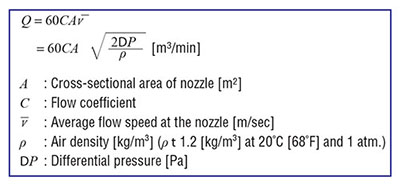The measurement of air flow – static pressure characteristics uses an auxiliary blower to control the pressure in chamber B, altering the pressure in chamber A. Thus, each point on the characteristics curve can be measured. Oriental Motor's measuring equipment is connected to a computer, providing extremely precise measurements in a short period of time.

Changes in Air Flow – Static Pressure Characteristics Using Two Fans

By using two fans featuring the same characteristics together, you can change the characteristics of the fans.

As shown in Figure 2, the maximum air flow is approximately twice as large using two fans.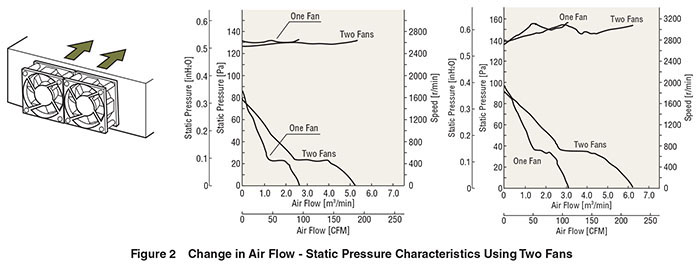Changes in the Air Flow - Static Pressure Characteristics with Installation of Accessories

When installing a fan in equipment, the safety and reliability of the overall apparatus can be significantly improved by attaching accessories, such as finger guards and filters. However, these parts produce air flow resistance, affecting fan characteristics and fan noise. This should be taken into account when selecting fans and accessories.

The graph in Figure 3 shows data regarding pressure loss caused by its accessories for a frame size 119 mm (4.69 in.) sq. fan. The filter causes the most significant pressure loss, while the finger guard causes little loss.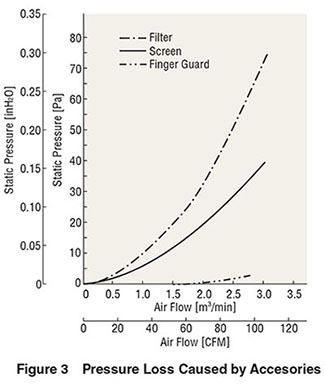The graphs in Figure 4 show how characteristics may change with installation of accessories, using the MU1225S-21 as an example.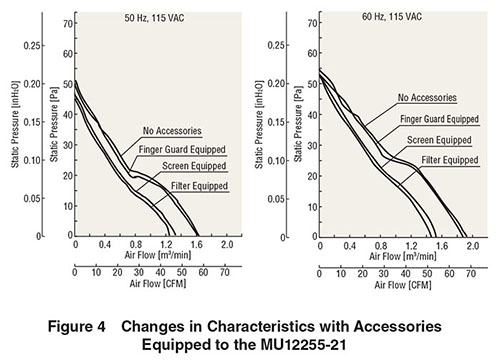As Figure 4 shows, the greater the pressure loss caused by accessories, the greater the reduction in air flow – static pressure characteristics.

Noise

What is Noise?

We generally refer to sounds that are unpleasant to us as "noise." In the case of fans, the rotation of the fan blades causes air pressure fluctuation and generates noise. The greater the change in air pressure, the louder the resulting noise will be.

Measurement of Noise

The noise level of Oriental Motor fans is measured in the A-weighted sound pressure level at a distance of 1 m (3.3 ft.) from the intake side (at a point above the center line of the intake side).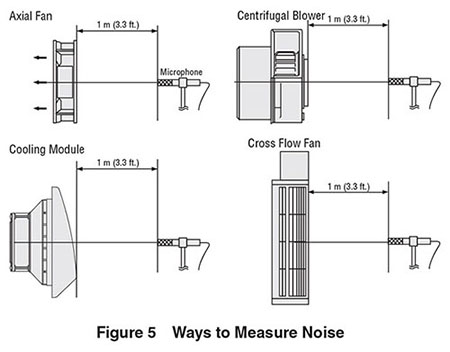Today, a comfortable life is well-established at home and work, thanks to advanced control systems. The equipment that functions as the core for such control has many electrical/electronic devices. An appropriate heat design is required for these devices to run uninterrupted 24 hours a day, 365 days a year. It also contributes to the reliability and longer operating life of the equipment, as well as reducing costs of the overall equipment. Oriental Motor provides products and service from the viewpoint of Thermal Management, which keeps customers' equipment at a consistent temperature and achieves energy savings used for the heat measures. Thermal Management not only achieves high reliability and long life of the product but also reduces noises caused by heat measures.

Composition of Noise

This section explains the noise level when using two fans, each of which produces 40 dB of noise. Noise is expressed in decibel units, and noise cannot be determined simply by adding individual noise levels. The value that expresses this combined noise is found by determining the energy of the noise and then using it to calculate the increase in sound pressure. The relationship between sound energy (J) and sound pressure (P) is expressed in the following formula: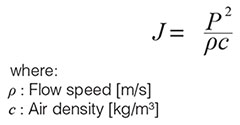Using the above formula, the noise level can be expressed in decibel unit as follows:In this formula the noise level is expressed in decibels based on the reference energy of J0. As the noise energy for n fans is n times that of a single fan, the sound pressure obtained by this formula will be: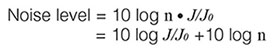In other words, when n fans are operated simultaneously, the increase in noise is equal to 10 log n [dB].

In this example, if two 40 dB fans (n = 2) are operated simultaneously, the increase in noise level is equal to 10 log 2 or 3 dB, and the combined noise level is 43 dB.

The following explains the combined noise level when a 40 dB fan and a 50 dB fan are operated simultaneously. Again, the combined noise level is not given by a simple arithmetic sum but is obtained as follows: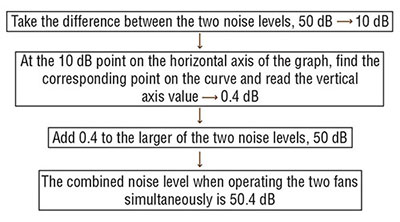If 40 dB of noise is combined with 50 dB, the resulting increase in noise level is only 0.4 dB. In other words, the noise level is always controlled by the larger of noise values, so it is important to suppress the noise of the fan producing greater noise.Distance and Noise

The noise level decreases as the distance from sound source increases. The decrease in noise level due to distance is given by the following formula: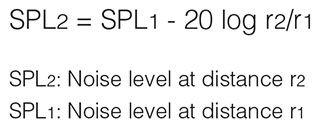In the following example the noise level at a distance of 2 m (6.6 ft.) from the intake side of fan, whose noise level is 40 dB at a distance of 1 m (3.3 ft.) from the intake side of fan, is calculated. Since r2 = 2 m (6.6 ft.), r1 = 1 m (3.3 ft.), and SPL1 = 40 dB, substituting in the formula gives: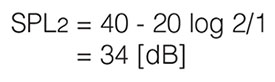Thus, at a distance of 2 m (6.6 ft.), the noise level decreases by 6 dB. The value 20 log r2/r1 in the above formula represents the ratio between two distances. Thus, if the values used above were 3 m (9.8 ft.) and 6 m (19.7 ft.), the result would have been the same.

Therefore, if the noise level at a certain distance is known, the noise level at another distance can be estimated.

Glossary

Distance and Noise

Noise level is expressed in decibel units (dB). When the noise level is expressed based on the linear scale, with the minimum level of noise audible to the human ear being 1, the maximum level of noise the human ear can withstand is expressed in such a substantial figure as 5 million. In contrast, if noise (sound pressure level) is expressed in decibels, thenTherefore, the range of sound pressure audible to the human ear can be conveniently expressed as 0 to 130 dB.

A-Weighted Sound Pressure Level

It is generally said that the audible range of the human ear is between 20 Hz and 20 kHz. Moreover, low frequency and extremely high frequency sounds are not disturbingly loud to the human ear.
For this reason, an accurate indication of loudness as perceived by the human ear cannot be achieved simply by measuring sound pressure without taking frequency into account. Therefore, measurements of the sound pressure level must be corrected according to frequency in order to accurately reflect the human perception of loudness. This corrected level is called the A-weighted sound pressure level.
Figure 6 compares the corrected measured values (A-weighted sound pressure level) with the uncorrected measured values (C-weighted sound pressure level).